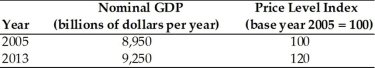• FAQ
• Contact/ Homework Answers / Economics / 41) If a nation's nominal GDP \$3,257 million and its
Not my Question
Flag Content

# Question

41) If a nation's nominal GDP is \$3,257 million and its price level is 132, then its real GDP is

A) \$4.05 million.

B) \$24.67 million.

C) \$4299.24 million.

D) \$2467.42 million.

42) Suppose nominal GDP in Canada increased by 5% in 2013.  Given this information, we know with certainty that

A) the aggregate price level in Canada increased in 2013.

B) real GDP in Canada increased in 2013.

C) both the aggregate price level AND real GDP increased in Canada in 2013.

43) Nominal GDP is calculated using

A) the market prices during the year under consideration.

B) the prices from some base year to adjust for price changes.

C) the average of prices from the three preceding years.

D) the prices from the preceding year.

44) Real GDP is

A) nominal GDP adjusted for depreciation.

B) nominal GDP adjusted for price changes.

C) nominal GDP adjusted for transfer payments.

45) Measuring total aggregate production in current dollars uses which of the following measures?

A) real personal income

B) real gross domestic product

C) nominal gross domestic product

D) nominal net domestic product

46) Assume that the nominal GDP for a given year is equal to \$12,400 billion and the GDP deflator equals 112. Real GDP for that year is approximately

A) \$13,888.0 billion.

B) \$12,512.0 billion.

C) \$11,071.4 billion.

D) \$12,228.1 billion.

47)  Constant dollars are dollars

A) corrected for general price level changes.

B) measured in terms of current-year prices.

C) issued by the U.S. Treasury with values that fail to change even in the face of inflation or deflation.

D) issued by the Federal Reserve with values that fail to change even in the face of inflation or deflation.

48) When one converts nominal GDP to real GDP, one takes into account which of the following?

A) changes in the population

B) changes in the quality of goods

C) changes in the distribution of income

D) none of the above

49) Measuring total aggregate production in constant dollars uses which of the following measures?

A) real personal income

B) real gross domestic product

C) nominal gross domestic product

D) nominal net domestic product50) Using the data in the above table, what is the real GDP for year 2005 (in billions of constant dollars)?

A) 9,250

B) 8,500

C) 7,708

D) 8,950

51) Using the data in the above table, what is the real GDP for year 2013 (in billions of constant dollars)?

A) 9,250

B) 8,500

C) 7,708

D) 8,950

52) Which of the following statements is true?

A) During periods of inflation, nominal GDP increases more rapidly than does real GDP.

B) Nominal values are expressed in constant dollars.

C) Real values are expressed in current dollars.

D) Increases in real GDP are evidence that the distribution of wealth is becoming more equal.

53) Is looking at changes in gross domestic product over time a good way to determine whether the people in a country are experiencing an increase in their standards of living? Why or why not?

## Solution 5 (1 Ratings )

Solved
Economics 2 Months Ago 6 Views